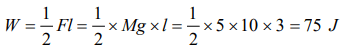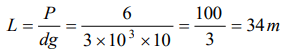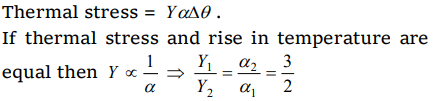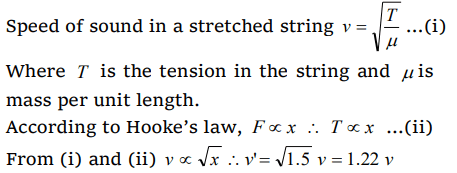## Mechanical Properties of Solids Questions and Answers Part-19

1. The work per unit volume to stretch the length by 1% of a wire with cross sectional area of 1mm2 will be. $\left[Y=9\times10^{11}N\diagup m^{2}\right]$
a) $9\times10^{11}J$
b) $4.5\times10^{7}J$
c) $9\times10^{7}J$
d) $4.5\times10^{11}J$

Explanation:2. When load of 5kg is hung on a wire then extension of 3m takes place, then work done will be
a) 75 joule
b) 60 joule
c) 50 joule
d) 100 joule

Explanation:3. A stretched rubber has
a) Increased kinetic energy
b) Increased potential energy
c) Decreased kinetic energy
d) Decreased potential energy

Explanation: Increased potential energy

4. Which of the following is true for elastic potential energy density
a) Energy density = $\frac{1}{2}\times strain \times stress$
b) Energy density = $\left(strain\right)^{2} \times volume$
c) Energy density = $\left(strain\right) \times volume$
d) Energy density = $\left(stress\right) \times volume$

Explanation: Energy density = $\frac{1}{2}\times strain \times stress$

5. A wire suspended vertically from one of its ends is stretched by attaching a weight of 200 N to the lower end. The weight stretches the wire by 1 mm Then the elastic energy stored in the wire is
a) 0.1 J
b) 0.2 J
c) 10 J
d) 20

Explanation:6.Wires A and B are made from the same material. A has twice the diameter and three times the length of B. If the elastic limits are not reached, when each is stretched by the same tension, the ratio of energy stored in A to that in B is
a) 2 : 3
b) 3 : 4
c) 3 : 2
d) 6 : 1

Explanation:7. An Indian rubber cord L metre long and area of cross-section A $metre^{2}$  is suspended vertically. Density of rubber is D $kg \diagup metre^{3}$   and Young's modulus of rubber is E $newton \diagup metre^{2}$   . If the wire extends by l metre under its own weight, then extension l is
a) $L^{2} Dg\diagup E$
b) $L^{2} Dg\diagup 2E$
c) $L^{2} Dg\diagup 4E$
d) L

Explanation: $L^{2} Dg\diagup 2E$

8. To break a wire, a force of $10^{6}N \diagup m^{2}$   is required. If the density of the material is $3\times10^{3}kg \diagup m^{3}$   , then the length of the wire which will break by its own weight will be
a) 34 m
b) 30 m
c) 300 m
d) 3 m

Explanation:9.Two rods of different materials having coefficients of linear expansion $\alpha_{1}$ ,$\alpha_{2}$ and Young's moduli $Y_{1}$ and $Y_{2}$ respectively are fixed between two rigid massive walls. The rods are heated such that they undergo the same increase in temperature. There is no bending of rods. If $\alpha_{1}:\alpha_{2}=2:3$   , the thermal stresses developed in the two rods are equally provided $Y_{1}:Y_{2}$  is equal
a) 2 : 3
b) 1 : 1
c) 3 : 2
d) 4 : 9